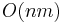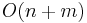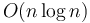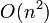# Algorithm

An algorithm is a (usually deterministic) well-defined procedure for solving a problem in a finite number of steps. The earliest algorithms, such as the Euclidean algorithm, were carried out by hand, but since the advent of modern computing technology they have come to be carried out largely by machines. Algorithms are ideally suited to solving problems using computers because, unlike humans, computers can only follow simple, precise, well-defined instructions. Together with data structures, algorithms form the foundation of computer science. Many algorithms have specific names, often after their inventors, but any computer program uses one or more algorithms, regardless of whether or not they are named.

## Process

### Design

Often the most difficult task involving algorithms, and the one requiring the most creativity, design is the synthesis of a new idea about how a problem may be solved. Algorithms, when first designed, generally exist in a highly abstracted form. For example, Euclid may have been thinking to himself when he thought of his algorithm for computing greatest common divisors, "What if I keep subtracting the smaller number from the larger one until they are the same"? Algorithms in this form cannot yet be directly executed by computers, nor is it immediately possible to determine how efficient they are. Nevertheless, the crux of the algorithm is developed in this stage, and its general structure emerges, including the general sequence of steps to perform (not yet simple enough to be executed by a computer) and the various sub-problems whose solutions will be incorporated into the solution of the problem at hand.

### Implementation

Implementation of an algorithm is the process by which it is translated from its abstract, newly designed form into machine language, which may be directly executed on a computer, and is, more or less, the proper name for what is often called simply programming, although in actual fact a programmer cannot implement without also performing design. This tends to be the longest stage of the design-implementation-analysis process. The abstracted form of the algorithm must first be refined and carefully fleshed out; in particular, the programmer must decide which data structures to use, and this often determines the efficiency of the resulting implementation. The algorithm must then be converted into a form that is readable by both humans and computers, the syntax of a programming language; the algorithm in this form is generally referred to as code, and writing code is colloquially referred to as coding. Finally, some combination of a compiler, assembler, and linker convert this syntax into a final concrete form, the machine code, which may be directly executed by a computer (often simply called a program). The testing phase follows; the code will be modified until the resulting program appears correct, sometimes using a tool called a debugger to help identify errors. The programmer may discover that the algorithm is incorrect and must be redesigned, or that the implementation does not accurately represent the algorithm and the code must be modified.

### Analysis

Main article: Analysis of algorithms

The analysis of an algorithm is its characterization in comparison to other algorithms intended to solve the same problem. Analysis may occur before or after implementation. During this stage, the programmer will almost always want to, at least, determine how efficient the program is; a program that use little time and memory to solve a given instance of a problem is more efficient than a program that takes a long time and uses lots of memory. The technique of asymptotic analysis is generally used to determine whether one algorithm is more efficient than another algorithm; this type of analysis corrects for differences in machine architecture. A more rigorous form of testing, known as verification, may also take place; this means proving mathematically that an algorithm is correct. Software engineers generally perform intuitive analyses of the algorithms they have designed and implemented to ensure that they are reasonably efficient. Computer scientists will often perform more detailed and mathematically rigorous analyses, publishing analysis along with the abstracted (and occasionally concrete) form of an algorithm. Analysis may allow the programmer to discover possible optimizations that allow the algorithm to be re-implemented in a more efficient form.

## Importance

Among factors that determine a program's efficiency, the choice of the underlying algorithm and the data structures used to implement it stand out above all others in importance. In the early days of computing, the increase in speed and power of computing machines, exponential as it was, was vastly outpaced by the growth in knowledge of algorithms, allowing large problems to be solved quickly on modestly powered hardware. This trend continues to this day.

Here is an example. Assume that the human genome numbers approximately 3.3×109 base pairs, and that the average gene contains 3×104 base pairs. Suppose that we wish to set up a web server that will allow biologists to search for genes in the human genome. Using a naive$O(nm)$ time algorithm for string searching, approximately 1014 operations would be required to search for a gene inside the human genome. If our web server can execute 109 operations per second, it may take up to 105 seconds to answer a single query (that is, more than a day). On the other hand, if we use the$O(n+m)$ Knuth–Morris–Pratt algorithm, only about 3.3×109 operations will be required, so the biologist will have her result in about three seconds.

## Types of algorithms

A deterministic algorithm is one that will always execute the same sequence of steps when presented with the same instance of a problem. If executed on the same machine, it will also take approximately the same amount of CPU time. In contrast, a nondeterministic algorithm is one with a random element to its execution: it may proceed through a different sequence of steps each time it is run, and it might not always take the same amount of time to solve the same instance on the same machine. There are two types of nondeterministic algorithms:

• A Monte Carlo algorithm always takes approximately the same amount of time to process inputs of the same size, but it may occasionally output a wrong answer.
• A Las Vegas algorithm always outputs the correct answer to any instance of the problem it solves, but may take much longer to process some inputs than others of the same size.

Most of the algorithms described on this wiki are deterministic, but nondeterministic algorithms are occasionally simpler to code and faster than their deterministic counterparts. An example is Welzl's algorithm, a Las Vegas algorithm for computing the minimum enclosing circle that almost always runs in linear time; it is far simpler than Megiddo's algorithm, which is deterministic and always solves this problem in linear time.

Furthermore, most of the algorithms described on this wiki are exact. This means that, if these algorithms run on theoretical machines capable of performing infinite-precision arithmetic, they will produce numerical output that is exactly correct or completely optimal. On the other hand, sometimes the simplest (or the only practical) method for solving a problem involves the use of an approximation algorithm. This is an algorithm which cannot necessarily produce an exact answer, even if we assume infinite-precision arithmetic is possible. Some approximation algorithms have bounded error, meaning that they will always produce an answer which is, for example, at least half as good the optimal answer. Others can be used to compute answers to within any degree of precision desired, but simply take longer and longer as we demand greater and greater precision. Algorithms based on binary search often fall into the latter category.

An asymptotically optimal algorithm is an algorithm which, in the limit of asymptotic analysis, is as efficient as any algorithm for solving the same problem can be. For example, it can be proven that no sorting algorithm can have better than$O(n \log n)$ worst-case or average-case time complexity. An algorithm that achieves this time bound, therefore, such as mergesort or heapsort, is asymptotically optimal. On the other hand, insertion sort is not asymptotically optimal, because it takes$O(n^2)$ time, but the faster$O(n \log n)$ exists. It is not always known what the time complexity of an asymptotically optimal algorithm is, or whether one even exists. (This is the case for the matrix multiplication problem.)

Algorithms are also categorized on the basis of the techniques they employ. For example, a recursive algorithm is one that operates by reducing a large instance of a problem to one or more "smaller" instances, which may be either solved directly or reduced to yet smaller instances. An example is the Euclidean algorithm. Recursive algorithms can often be made more efficient with memoization or by converting them into dynamic algorithms, such as the classic longest increasing subsequence algorithm, which start by solving the smallest instances and using these build up the solutions to larger instances. If a recursive algorithm is tail-recursive, it may be implemented using a simple iterative scheme in an imperative programming language. A recursive or iterative algorithm that solves an optimization problem by making a series of locally optimal ("short-sighted") selections is known as a greedy algorithm (an example is Dijkstra's algorithm).

In addition, algorithms can be categorized on the basis of the problems they are intended to solve. For example, an algorithm that solves the sorting problem is called a sorting algorithm, an algorithm that finds shortest paths is called a shortest path(s) algorithm, and so on; a graph-theoretic algorithm is any algorithm that solves any problem in graph theory, and so on.